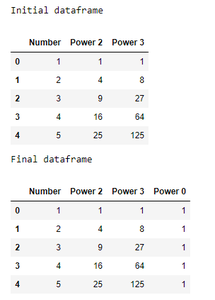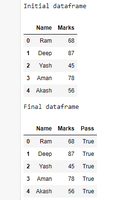# Add column with constant value to pandas dataframe

Prerequisite: Pandas

In this article, we will learn how to add a new column with constant value to a Pandas DataFrame. Before that one must be familiar with the following concepts:

• Pandas DataFrame : Pandas DataFrame is two-dimensional size-mutable, potentially heterogeneous tabular arrangement with labeled axes (rows and columns). A Data frame may be a two-dimensional arrangement , i.e., data is aligned during a tabular fashion in rows and columns. Pandas DataFrame consists of three principal components, the data, rows, and columns.
• Column in DataFrame : In Order to pick a column in Pandas DataFrame, we will either access the columns by calling them by their columns name. Column Addition: so as to feature a column in Pandas DataFrame, we will declare a replacement list as a column and increase a existing Dataframe.
• Constant : A fixed value. In Algebra, a continuing may be a number on its own, or sometimes a letter like a, b or c to face for a hard and fast number. Example: in “x + 5 = 9”, 5 and 9 are constants.

## Approach

1. Import Library
2. Load or create a dataframe
3. Add column with constant value to dataframe

To understand these above mentioned steps, lets discuss some examples :

Example 1: (By using Pandas Series)

## Python3

 `# import packages ` `import` `pandas as pd ` `import` `numpy as np ` ` `  `# create dataframe ` `df ``=` `pd.DataFrame({``'Number'``: {``0``: ``1``, ``1``: ``2``, ``2``: ``3``, ``3``: ``4``, ``4``: ``5``}, ` `                   ``'Power 2'``: {``0``: ``1``, ``1``: ``4``, ``2``: ``9``, ``3``: ``16``, ``4``: ``25``}, ` `                   ``'Power 3'``: {``0``: ``1``, ``1``: ``8``, ``2``: ``27``, ``3``: ``64``, ``4``: ``125``}}) ` ` `  `# view dataframe ` `print``(``"Initial dataframe"``) ` `display(df) ` ` `  ` `  `# adding column with constant value ` `df[``'Power 0'``] ``=` `pd.Series([``1` `for` `x ``in` `range``(``len``(df.index))]) ` ` `  `# view dataframe ` `print``(``"Final dataframe"``) ` `display(df) `

Output :Example 2: (As static value)

## Python3

 `# import packages ` `import` `pandas as pd ` `import` `numpy as np ` ` `  `# create dataframe ` `df ``=` `pd.DataFrame({``'Name'``: {``0``: ``'Ram'``, ``1``: ``'Deep'``, ``2``: ``'Yash'``, ``3``: ``'Aman'``, ``4``: ``'Akash'``}, ` `                   ``'Marks'``: {``0``: ``68``, ``1``: ``87``, ``2``: ``45``, ``3``: ``78``, ``4``: ``56``}}) ` ` `  ` `  `# view dataframe ` `print``(``"Initial dataframe"``) ` `display(df) ` ` `  ` `  `# adding column with constant value ` `df[``'Pass'``] ``=` `True` ` `  `# view dataframe ` `print``(``"Final dataframe"``) ` `display(df) `

Output :Whether you're preparing for your first job interview or aiming to upskill in this ever-evolving tech landscape, GeeksforGeeks Courses are your key to success. We provide top-quality content at affordable prices, all geared towards accelerating your growth in a time-bound manner. Join the millions we've already empowered, and we're here to do the same for you. Don't miss out - check it out now!

Previous
Next